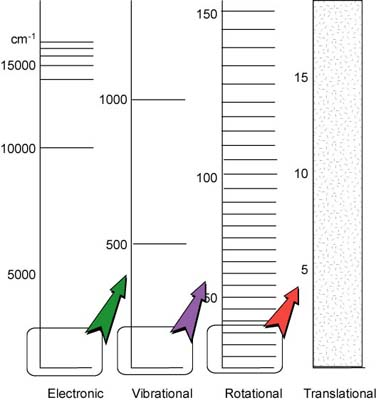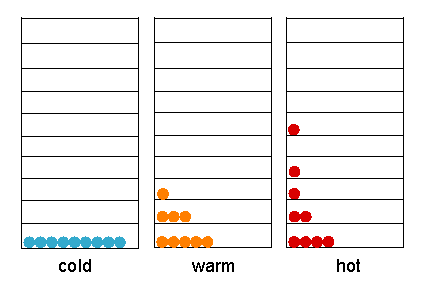### Introduction

An understanding the properties of matter depends on the knowledge about its energy and its coordinates in space and time, i.e., its conformation and dynamic properties. There are several important things we need to know:

• The physical and chemical properties can be described in detail by Schrodinger's wave function (Y).
• The distribution of a particle in space is given by the square of its wave function |Y|2. This leads an understanding of orbitals.
• Energy states are quantized; thus any system has certain characteristic energy values or levels.

### Energy levels

The energy levels represent the characteristic states of the molecule. The properties of the characteristic states are related to the identity of the molecules, to the molecular structure, and to the energetics of any chemical processes that the molecules may undergo.

For convenience, the ground state is defined as the state of the lowest energy. The ground state is the state that becomes progressively more occupied by the molecule as matter is cooled toward absolute zero. States of higher energy are called excited states.

If two or more states of the molecule have the same numerical value of energy, then they are said to be degenerate. This degeneracy may be removed by the effect of some external influence, e.g. electric or magnetic fields.

### Classification of energies

For most purposes, it is convenient to treat a molecula as if it possesses several distinct reservoirs of energy. The total energy is then given by

Etotal = Etranslation + Erotation + Evibration + Eelectronic + Eelectron spin orientation + Enuclear spin orientation

Each E in the equation represents the appropriate energy as indicated by its subscript. In soultioon, a molecule can obvious translate, rotate, and vibrate. The energies associated with each of these are quantized. Probably more familiar is the idea that the electrons have certain allowed energies, which are defined by orbitals.

### Probing different energies with different ranges of electromagnetic radiation

 Although every type of energy is quantized, the separation between neighboring translational energy is so small that for pratical purpose we can disgard the quantization of the translational energy. The separation between energy levels associated with the orientations that a nuclear or electron spin can take in a magnetic field are also very small (10-3 J*mol-1), but they are very precise because their life-times are long. Three or four orders of magnitude greater are the separations between the energy levels associated with the different rates of rotation of a moloecule (10 J*mol-1). The separation between electronic energy levels is even greater (105-106 kJ*mol-1). -An illustration of the relative separation of the energy of atoms and molecules for the different types of energy. The translational energy levels are essential continuous.

### Population of energy levels

At any finite temperature, the molecules will be distributed among the energy levels availabe to them because of thermal agitation. The exact distribution will depend on the temperature (thermal energy) and on the separation between energy levels (DE) in the energy ladder. At a given temperature, the number of molecules in an upper state (nupper) relative to the lower state (nlower) is given by Boltzmann distribution law

nupper / nlower = exp[-DE / kT]

where k is the Boltzmann constant (k = 1.38*10-23 J*K-1). When DE applies to 1 mol, the term on the right becomes exp[-DE / RT], where R is the gas constant (R = 8.31 J*mol-1).The dependence of the population of energy levels on temperature.

It is clear that when DE << kT, exp[-DE / kT] approaches to1. The number of molecules in the upper and lower levels is then equal. Conversely, when DE >> kT, nupper is neglible with respect to nlower.

Q: Calculate the ratio of molecules in the upper to those in the lower energy level when the separation between these is as follows. Assume the temperature is 300 K. Three type of energy involved are shown.

1. 11.9 J*mol-1 (rotational)
2. 11.9 kJ*mol-1 (vibrational)
3. 119 kJ*mol-1 (electronic)

solution.....

[BACK]